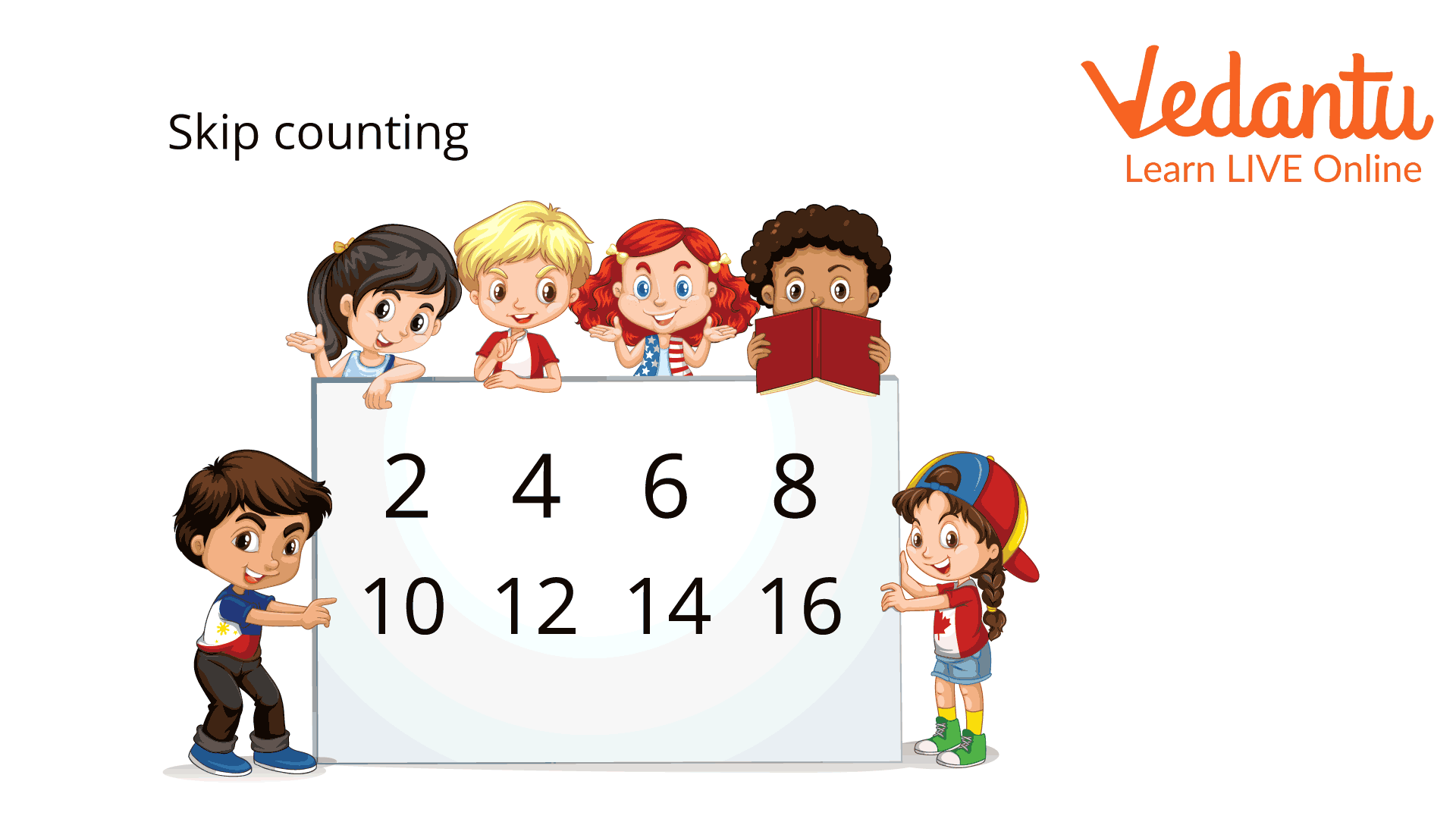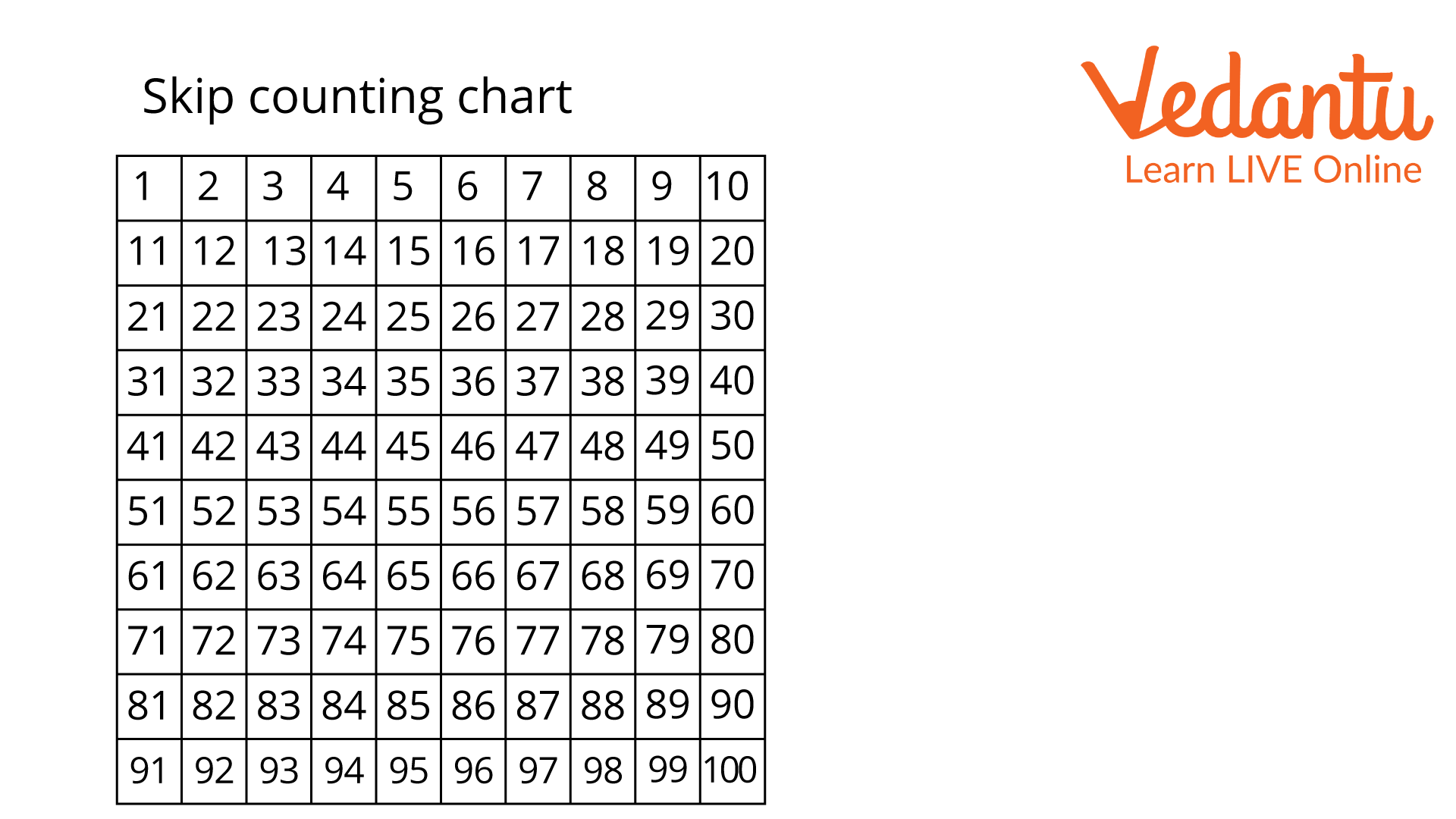Courses
Courses for Kids
Free study material
Offline Centres
More

# Skip Counting by 1Last updated date: 27th Nov 2023
Total views: 98.1k
Views today: 2.98k## How to Skip Count by 1?

Skip counting is the method of counting forward by numbers like 1, 2, 3, and so on. In other words, by doing this, we skip over a particular number. For example, when we skip count by 3, we start from 0 and skip the next number to go to the third number, i.e., 3. Therefore, skip counting by 1 means skipping through 1.Skip counting

## What is Skip Counting by 1?

Skip counting by 1, as explained above, means skipping through 1. If we start from 0, the next number will be 1 itself. If we continue, the next number will be 1, 2, 3, 4, 5, and so on, as shown in the image below.Skip counting by 1 chart

Skip counting is useful in multiplication tables because we can skip numbers to find the multiple of a number. For example, if you want to know the multiple of 6 in a multiplication table of 1, you can skip count by one 6 times. Thus, the result will be 6. We can use skip counting by 1 to 100 worksheets like the one given above for practice.

## Types of Skip Counting

Skip counting has two types: forward skip counting and backward skip counting.

• ### Forward Skip Counting

When we perform "forward skip counting," we count numbers in the forward direction. For example, if we had to perform forward skip counting by 1, and we began with 0, then the next number would be 1. If we continue forward counting, the number will be 1, 2, 3, 4, and so on.

• ### Backwards Skip Counting

When we perform backwards skip counting, we count numbers in the backward direction. For example, if you skip count backwards by 1 starting from the number 9, the next number would be 8. If we continue skip-counting backward, the numbers will be 8, 7, 6, and so on.

## Skip Counting by 1 Worksheet

1. Ravi has 20 marbles. How many times will he have to skip count by one?

Ans: Since the total number of marbles is 20, Ravi would have to skip count by 1, 20 times to get the total number of marbles. He can start by counting 1, 2, 3, 4,... 20.

2. Sneha has 10 candies. How many times will she need to perform a skip count by 1?

Ans: The total number of candies is 10. Sneha would need to skip count by 1, 10 times to get the total number of candies. She can skip count by counting 1, 2, 3, 4, 5, and so on, up to 10.

3. How many times will you need to skip count by 1 while climbing 15 stairs?

Ans: Since there are 15 stairs, while climbing the first one, you skip counting by 1. As you skip count by 1, the numbers will be 1, 2, 3, 4,... 15.

## Conclusion

So, today you have learned about skip counting and skip counting by 1 type, i.e., forward and backward skip counting, with some examples. Now you can count anything easily by using this method. Following similar rules, you can skip counting higher numbers like 2 and 3.

## FAQs on Skip Counting by 1

1. Where can we use skip counting?

Skip counting is very useful for children while learning multiplication tables. If a child wants to know the result of 5 x 6, they can skip the count by 5 or 6 times to get the result, which will be 30. As the child learns one-digit skip, we can introduce skip counting by higher numbers.

2. How do we introduce skip counting to children?

To teach a child to skip count, we start with 1. As the child learns, we progress to 2, and so on. This way, the child will skip count by 10 or 20. We can also provide them a skip counting by 1 worksheet.

3. How do you make skip-counting fun for kids?

To learn, you can perform some skip counting by one activity, like playing with the kids while climbing stairs, giving them candies, and introducing skip counting, or giving them a chart with numbers like a snake and ladder game and introducing skip counting where they move their token to the said number by skipping counting.